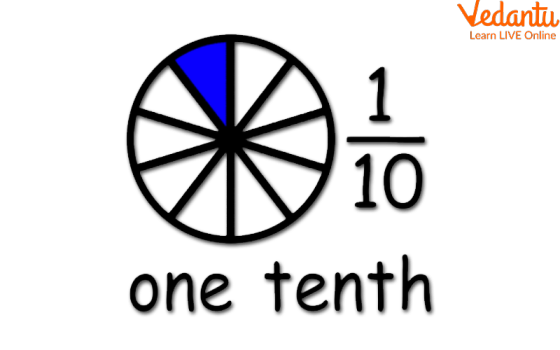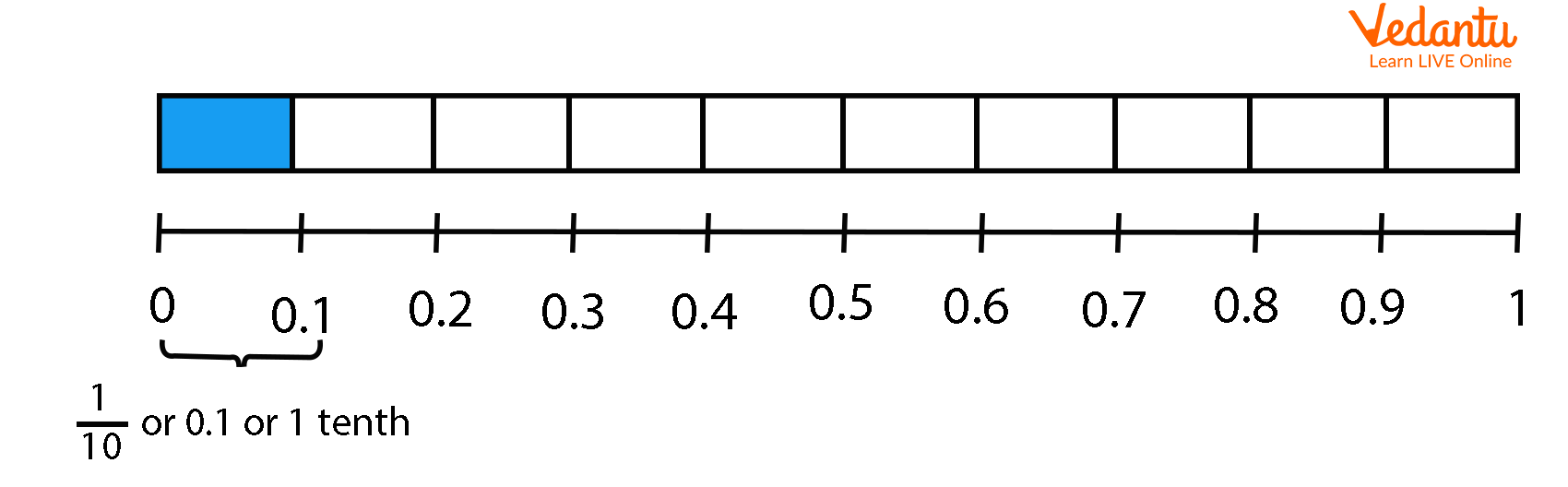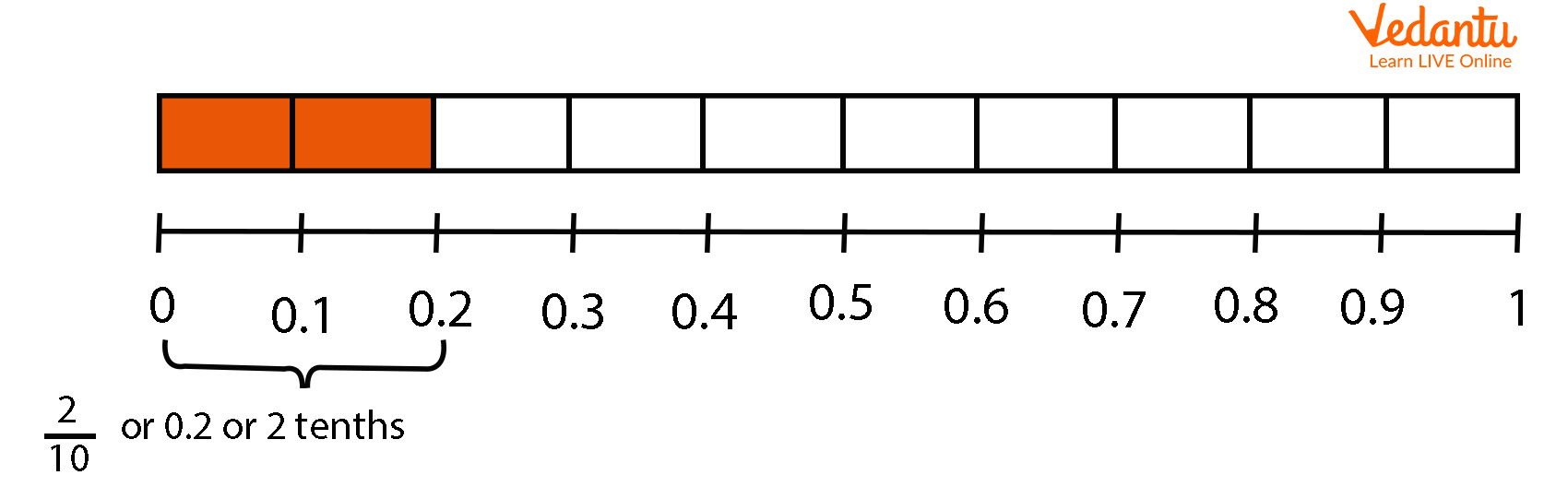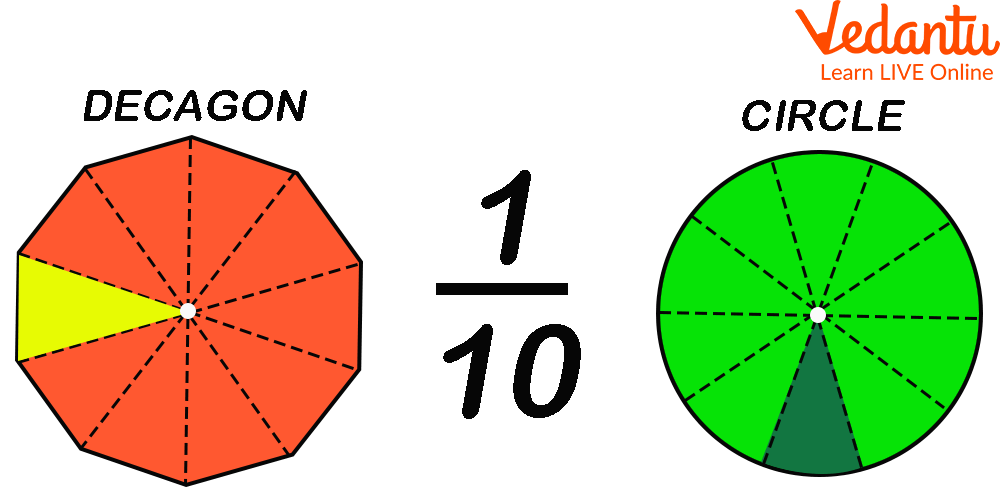Courses
Courses for Kids
Free study material
Offline Centres
More

# One-Tenth in Decimal FormLast updated date: 04th Dec 2023
Total views: 152.4k
Views today: 4.52k## How to Write One-Tenth in Decimal Form?

Do you know what is one-tenth? Suppose, you have 10 chocolates and you gave one to your little sister. That means you gave one-tenth of your chocolates to your sister. One-tenth is basically one part out of ten equal parts.

To write one-tenth in decimal form, first, we need to understand the meaning of one and one-tenth. Then, we will write one-tenth in decimal form.One-tenth

## What is One?

One is a number that represents the whole quantity. It is written as ‘1’.

## What is One-tenth?

One is written in ten equal parts:What is One-tenth

In the above-given figure, 1 is divided into 10 equal parts. The value of each part is equal to $\frac{1}{10}$ or 1-tenth.

So we can say that when one is divided into ten equal parts, then each part of the whole is equal to 0.1 and is called one-tenth.

## One-tenth in Decimal Form

From the above-given figure, it is clear that if we divide ‘1’ into ten equal parts, then each part has a value of 0.1. Therefore, one-tenth is equal to 0.1.One-tenth in Decimal Form

In the above figure, if we take two parts of the whole, it will form $\frac{2}{10}$ or 2 tenth or 0.2, and so on.

Now, let us consider some more examples to understand the value of one-tenth.One-tenth of a Circle and Decagon

Here, a circle is divided into ten wedges. All the wedges are equal and one-tenth of a circle. Similarly, if a decagon has 10 edges, all edges drawn are of equal size. Decagon is divided into 10 equal parts that form 10 equivalent triangles and each part is equal to one-tenth or decimal equivalent 0.1.

## How to Get One-tenth of Any Number?

To get the one-tenth of a number, multiply it with $\frac{1}{10}$.

Some Examples Related to One-tenth of Numbers in Decimal Forms

Example 1: What is the one-tenth of 20?

Solution: One-tenth of 20 = 20 x $\frac{1}{10}$= 2

Example 2: What is the one-tenth of 100?

Solution: One-tenth of 100 = 100 x $\frac{1}{10}$ = 10

Example 3: What is the one-tenth of 75?

Solution: One-tenth of 75 = 75 x $\frac{1}{10}$= 7.5

Example 4: What is the one-tenth of 0.25?

Solution: One-tenth of 0.25 = 0.25 x $\frac{1}{10}$=0.025

## Percentage Value of One-tenth

As we know one-tenth is equal to $\frac{1}{10}$, so we can find its percentage value like any other fractions. To find its percentage value, we will multiply it by 100 and add a % sign with it.

Therefore, the percentage value of one-tenth = $\frac{1}{10}$× 100% = 10 %.

## Conclusion

In this article, we have learned the meaning of one-tenth, and how to write one-tenth of any number in decimal form. Understanding this concept will help you solve other sums on fractions, decimals, and percentages.

## FAQs on One-Tenth in Decimal Form

1. What do you understand by the term one-tenth?

One-tenth is the one part of equivalent 10 parts. If we divide a number or a figure into 10 equal parts, then each part is one-tenth of a whole.

One-tenth = $\frac{1}{10}$ = 0.1

One-tenth of any number, say ‘a’, could be evaluated as:

One-tenth of ‘a’ = a x $\frac{1}{10}$

2. What is the percentage value of one-tenth?

The percentage value of one-tenth, i.e., $\frac{1}{10}$ is 10 %.

This value is evaluated by simply converting a fractional number to a percentage.

The percentage value of one-tenth = $\frac{1}{10}$× 100% = 10 %

Therefore, we can say that

$\frac{1}{10}$ = 0.1 = 10 %